# 为开发游戏做准备—判断线段相交(JavaScript 版本)<head>
<meta charset="UTF-8">
<meta http-equiv="X-UA-Compatible" content="IE=edge">
<meta name="viewport" content="width=device-width, initial-scale=1.0">
<title>Segment Intersection</title>
<style>
body {
margin: 0;
overflow: hidden;
}
</style>
<body>
<canvas id="mCanvas"></canvas>
</body>
</html>

### 定义画布

mCanvas.width = window.innerWidth;
mCanvas.height = window.innerHeight;
const ctx = mCanvas.getContext('2d');

### 绘制线段

const A = { x: 200, y: 150 };
const B = { x: 150, y: 260 };
const C = { x: 50, y: 100 };
const D = { x: 260, y: 200 };

ctx.beginPath();
ctx.moveTo(A.x, A.y);
ctx.lineTo(B.x, B.y);
ctx.moveTo(C.x, C.y);
ctx.lineTo(D.x, D.y);

ctx.stroke();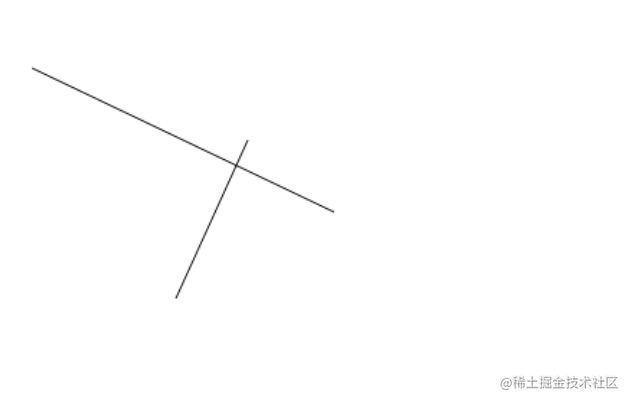### 绘制标注

function drawDot(point, label) {
ctx.beginPath();
ctx.fillStyle = "white";
ctx.arc(point.x, point.y, 10, 0, Math.PI * 2);
ctx.fill();
ctx.stroke();
ctx.fillStyle = "black";
ctx.textAlign = "center";
ctx.textBaseline = "middle";
ctx.font = "bold 14px Arial";
ctx.fillText(label, point.x, point.y);
}

ctx.textAlign = "center";
ctx.textBaseline = "middle";

drawDot(A, "A");
drawDot(B, "B");
drawDot(C, "C");
drawDot(D, "D");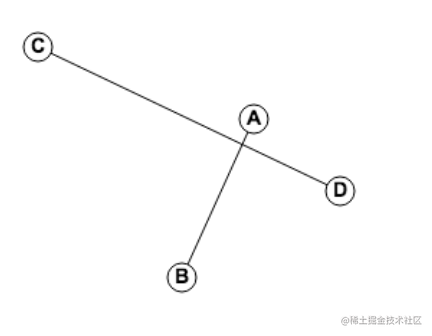### 绘制线段上的插值

const M = {
x: lerp(A.x,B.x,0.5),
y: lerp(A.y,B.y,0.5)
}

function lerp(A,B,t){
return A + (B-A)*t
}

drawDot(M,"M")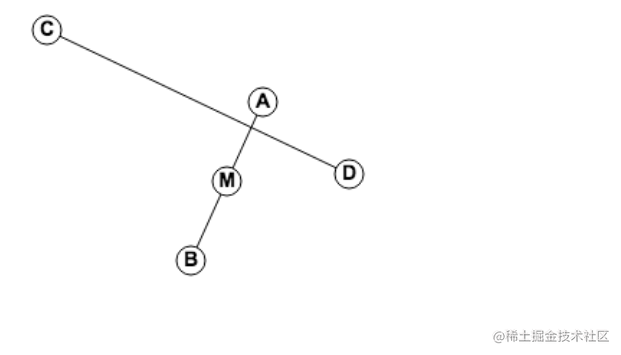### 添加动画

let t = 0;
animate();
function animate() {
ctx.clearRect(0, 0, mCanvas.width, mCanvas.height);
ctx.beginPath();
ctx.moveTo(A.x, A.y);
ctx.lineTo(B.x, B.y);
ctx.moveTo(C.x, C.y);
ctx.lineTo(D.x, D.y);

ctx.stroke();

drawDot(A, "A");
drawDot(B, "B");
drawDot(C, "C");
drawDot(D, "D");

const M = {
x: lerp(A.x, B.x, t),
y: lerp(A.y, B.y, t)
}
drawDot(M, "M");
t += 0.02;

requestAnimationFrame(animate);
}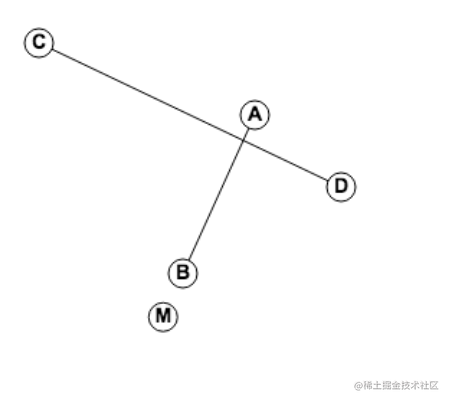drawDot(M, "M", t < 0 || t > 1);

function drawDot(point, label, isRed) {
ctx.beginPath();
ctx.fillStyle = isRed ? "red" : "white";
...
}

$I_x = A_x + (B_x - A_x)t = C_x + (D_x - C_x)u\\ I_y = A_y + (B_y - A_y)t = C_y + (D_y - C_y)u\\$

$A_x - C_x + (B_x - A_x)t =(D_x - C_x)u$

$A_y + (B_y - A_y)t = C_y + (D_y - C_y)u$

$(A_y - C_y)+ (B_y - A_y)t = (D_y - C_y)u$

$(A_y - C_y)(D_x - C_x)+ (B_y - A_y)(D_x - C_x)t = (D_y - C_y)(D_x - C_x)u$
$(D_x - C_x)u = A_x - C_x + (B_x - A_x)t$

$(D_x - C_x)u$ 带入到 $(A_y - C_y)(D_x - C_x)+ (B_y - A_y)(D_x - C_x)t = (D_y - C_y)(D_x - C_x)u$ 就可以求得 $t$

$t = \frac{(D_x - C_x)(A_y - C_y) - (D_y - C_y)(A_x - C_x)}{(D_y - C_y)(B_x - A_x) - (D_x - C_x)(B_y - A_y)}$

function getIntersection(A, B, C, D) {
const top = (D.x - C.x) * (A.y - C.y) - (D.y - C.y) * (A.x - C.x)
const bottom = (D.y - C.y) * (B.x - A.x) - (D.x - C.x) * (B.y - A.y)

const t = top / bottom;
return {
x: lerp(A.x, B.x, t),
y: lerp(A.y, B.y, t)
}
}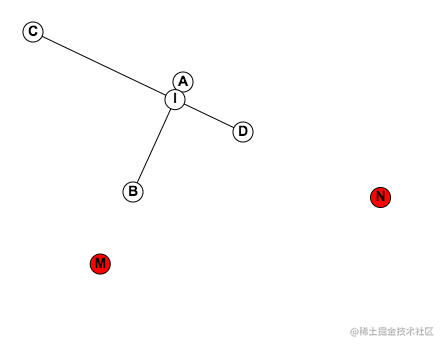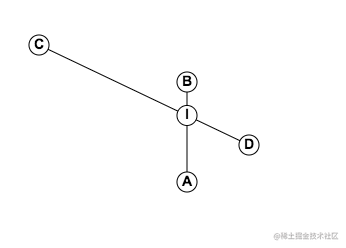### 验证

const mouse = {x:0,y:0};
document.onmousemove=(event=>{
mouse.x = event.x;
mouse.y = event.y;
});

function animate() {
A.x = mouse.x;
B.x = mouse.x;

...
}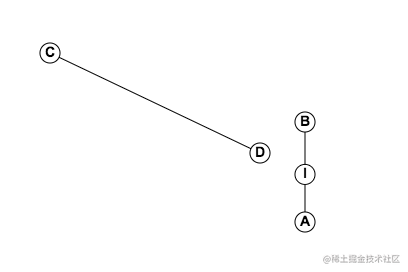let angle = 0;

let angle = 0;
document.onmousemove=(event=>{
mouse.x = event.x;
mouse.y = event.y;
})

// let t = 0;
animate();
function animate() {

angle+=0.02;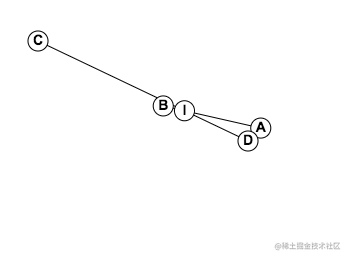### 优化代码

function getIntersection(A, B, C, D) {
const top = (D.x - C.x) * (A.y - C.y) - (D.y - C.y) * (A.x - C.x)
const bottom = (D.y - C.y) * (B.x - A.x) - (D.x - C.x) * (B.y - A.y)

if(bottom != 0){
const t = top / bottom;
if(t>=0 && t<=1){
return {
x: lerp(A.x, B.x, t),
y: lerp(A.y, B.y, t),
offset:t
}
}
}
return null
}